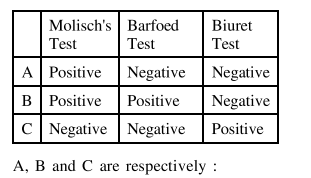# A, B and C are three biomolecules. The results of the tests performed on them are given below:

Question:

A, B and C are three biomolecules. The results of the tests performed on them are given below:1. $\mathrm{A}=$ Glucose, $\mathrm{B}=$ Fructose, $\mathrm{C}=$ Albumin

2. $\mathrm{A}=$ Lactose, $\mathrm{B}=$ Fructose, $\mathrm{C}=$ Alanine

3. $\mathrm{A}=$ Lactose, $\mathrm{B}=$ Glucose, $\mathrm{C}=$ Alanine

4. $\mathrm{A}=$ Lactose, $\mathrm{B}=$ Glucose, $\mathrm{C}=$ Albumin

Correct Option: , 4

Solution:

Alanine does not show Biuret test because Biuret test is used for deduction of peptide linkage \& alanine is amino acid.

Albumine is protein so have paptide linkage so it gives positive Biuret test.

Positive Barfoed test is shown by monosaccharide but not disaccharide. Positive Molisch's test is shown by glucose.